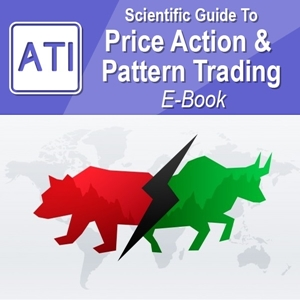•### Scientific Guide to Price Action and Pattern Trading

Subtitle: Wisdom of Trend, Cycle, and Fractal Wave

Author: Young Ho Seo

Financial Trader, Engineer, and Quantitative Developer

Publication Date: 7 July 2017

List of our E-Book Distributor

Just click link below to purchase our book from other Reputable Distributors. Use one of this reliable distributor to buy our eBooks.

Also you can visit this link to view all the distributors of our EBook:

Introduction to Five Regularities in the Financial Market 8

1. Introduction to Technical Analysis 9
2. Introduction to Charting Techniques 24
3. The Five Regularities in the Financial Market 34
4. Random Process 47
5. Stationary Process (No Trend) 52
6. Equilibrium Process (Trend) 59
7. Wave Process (Cycle) 67
8. Fractal-Wave Process 75
9. Equilibrium Wave Process (Trend and Cycle) 89
10. Equilibrium Fractal-Wave Process (Trend and Fractal Wave) 97
11. Choice of Trading Strategy and Price Patterns 120
12. Peak Trough Transformation to Visualize Equilibrium Fractal Wave 129
13. Using Equilibrium Fractal Wave Index to Select Your Trading Strategy 144
14. Appendix-Equilibrium Fractal Wave Derived Patterns 160
15. Appendix-Fractal Dimension Index for Financial Market 167
16. Appendix-General 171
17. References 177

Price Action and Pattern Trading 185

Overview on Practical Trading with the Fifth Regularity 186

1. Fibonacci Analysis and Golden Ratio 190

2. Support and Resistance 202
2.1 Horizontal Support and Resistance 203
2.2 Diagonal Support and Resistance 211
2.3 Identification of Support and Resistance with the Template and Pattern Approach 221

3. Trading with Equilibrium Fractal Wave 228
3.1 Introduction to EFW Index for trading 228
3.2 Trading with the Shape ratio of Equilibrium Fractal Wave 233
3.3 Introduction to Equilibrium Fractal Wave (EFW) Channel 238
3.4 Practical Trading with Equilibrium Fractal Wave (EFW) Channel 245
3.5 Superimposed Equilibrium Fractal Waves 252
3.6 Combining the Shape Ratio Trading and (EFW) Channel 258

4. Harmonic Pattern 262
4.1 Introduction to Harmonic Pattern 262
4.3 Pattern Completion Interval (PCI) 275
4.4 Potential Reversal Zone (PRZ) 284
4.5 Potential Continuation Zone (PCZ) 288
4.6 Practical Trading with Harmonic Patterns 292

5.1 Introduction to the Wave Principle 294
5.2 Scientific Wave Counting with the Template and Pattern Approach 297
5.3 Impulse Wave Structural Score and Corrective Wave Structural Score 311
5.4 Channelling Techniques 316

6. Triangle and Wedge Patterns 320
6.1 Introduction to Triangle and Wedge patterns 320
6.2 Classic Perspective of Triangle and Wedge Patterns 323
6.3 Diagonal Support and Resistance Perspective of Triangle and Wedge Pattern 331
6.4 Elliott Wave Perspective of Triangle and Wedge Pattern 334
7. References 338

1. Risk Management 340
1.1 Various Risks in Trading and Investment 341
1.2 Position Sizing Techniques 346
1.4 Breakeven Success Rate 352
1.6 Compounding Profits 358
1.7 Trading Performance and Cost Metrics 358
2. Portfolio Management 363
2.1 Combining Different Trading Strategy 363
2.2 Hedging 372
2.3 Portfolio Diversification 374
3. References 377

X3 Pattern Notation for Tradable Patterns in the Financial Market 380

1. Overview on Tradable Patterns in the Financial Market 381
2. Why Do We Need New Pattern Notation X3? 381
3. How the New Notation X3 is different from other Approaches 383
4. Definition of EFW, Shape Ratio and Momentum Ratio 383
5. Scientific Lag Notation for Shape Ratio and Momentum Ratio 387
6. Closing Shape Ratio to Describe the Structure of Pattern 391
7. Factored Momentum Ratio to Describe Structure of Pattern 396
8. Converting Number of Points to Number of EFWs 399
9. X3 Pattern Notation with Name, Structure and Ideal Ratios 400
9.1 Pattern with 1 EFW (3 points) Examples 401
9.2 Pattern with 2 EFWs (4 points) Examples 403
9.3 Pattern with 3 EFWs (5 points) Examples 406
9.4 Pattern with 4 EFWs (6 points) Examples 409
9.5 Pattern with 7 EFWs (9 points) Examples 410Subscribe
Notify of